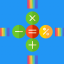Math Puzzle and quiz : Increase Mathematical Skill - Slunečnice.cz
Hlavní navigace

#Math Puzzle and quiz : Increase Mathematical Skill 1.0

3

Pro hodnocení programu se prosím nejprve

Staženo 0 ×
Zdarma

## Sdílet

Do you want to know mathematical tricks and forget about calculator in your daily live? This math trainer app will help you to get mathematical skills and learn psychic math. Learn and train math in cool mathematical games!  Math puzzles increases your mathematical thinking.

The application will be useful for all ages: - students and kids - to master the basics of mathematics and arithmetic, learn multiplication table, prepare for math tests and exams, learn how to fold, subtract, multiply, divide.

- adults - to keep their mind and brain in good shape, improve results in iq test, quickly solve logic games.

-This Maths game contains 4000+ challenging brain teasers. Each brain teaser offers a different and unique challenge which forces the user to think in unconventional way.

- Easy to understand UI.

Rules:
Each match Quiz 1000 level and each level 20 question if you passes first level then automatically unlock next level.
Result screen display your result. You are passed or fail if you are passed then display your start also.
Minimum 50% answers right than you are able to go to next level means require compulsory 50 % mark of each puzzle or quiz.

There are four type puzzle and quiz available.
1) Addition maths puzzle and quiz.
2) Subtraction maths puzzle and quiz.
3) Multiplication maths puzzle and quiz.
4) Division maths puzzle and quiz.

■ Learning: - Study mental calculation tricks for addition, subtraction, multiplication, division.
■ Brain Training Apps Activate the brain through mental arithmetic with brain training apps. Begin mental gymnastics once a day!
■ Application Features Have a great time playing with family and friends. Play

1.0

• #### Poslední aktualizace od vývojáře

25. 12. 2017 2017-12-25

0 ×

• Hádanky
• #### Podporované jazyky

• Angličtina
Zobrazit více
3

Pro hodnocení programu se prosím nejprve

Staženo
0 ×

TIP: Stahují se vám programy pomalu? Změřte si rychlost svého internetového připojení.